#### New products

» Do not allow new products at this time.

#### Recent posts

• #####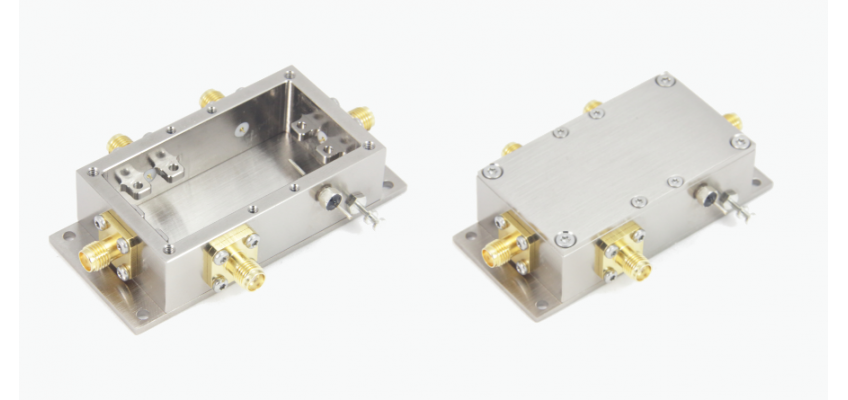RF Enclosure Mini-Extended-FX: a...
Sep 15, 2021 4100
• #####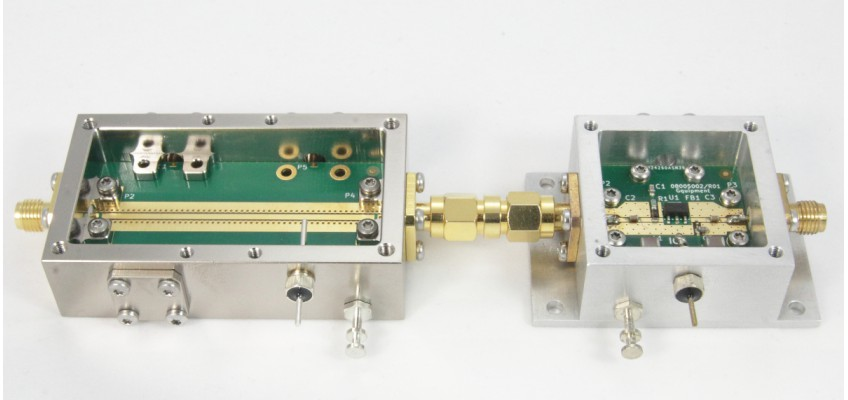Aluminum RF and mmWave Enclosures
Aug 22, 2021 4923
• #####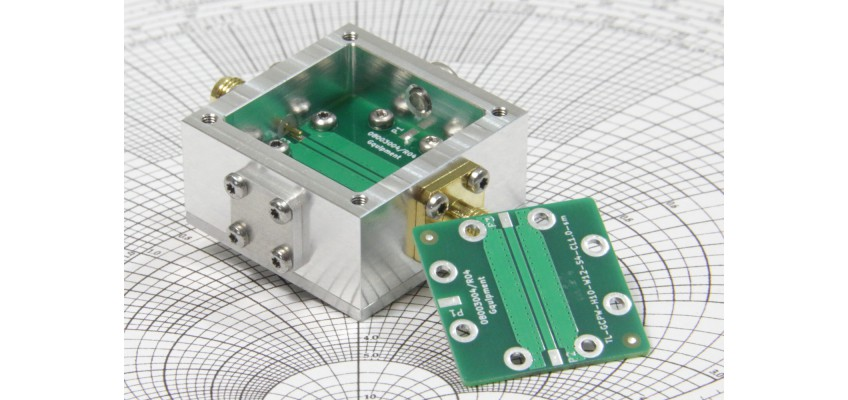SMA connector performance in the...
Feb 6, 2021 6359
• #####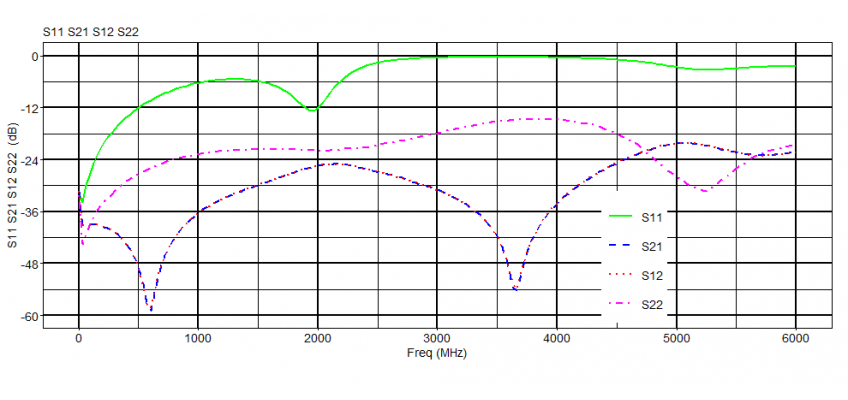Online S-parameter viewer
Nov 15, 2020 8989
• #####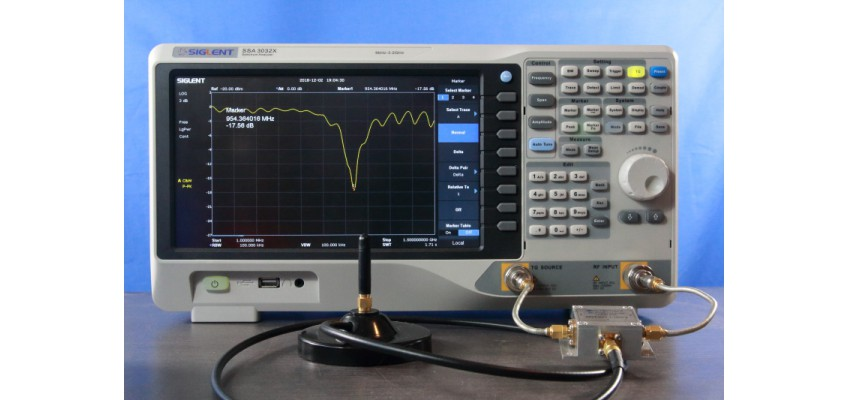Everything You Should Know About...
May 13, 2019 28778

Resistive - 2R config

Resistive power splitters that use a two-resistor configuration (2R) should be used only for applications where the output power values are mathematically divided or for power-leveling applications in which the splitter is part of a feedback loop. For all other applications, use a 3R power splitter.

What makes a 2R splitter unique is the fact that the power is always equal at both output terminals. Therefore, any power reflected at one port will also be emanated at the other output port. This makes this kind of splitter the only correct choice for the above-mentioned applications.

An example of an application in which the output values are mathematically divided is a setup where one output of the splitter is used as a reference value A and the other port feeds some DUT which in response outputs a signal B. Then, the A and B signals are measured with an analyzer that computes the gain of the DUT by dividing B by A.

In power-leveling applications, a feedback process is used to set the power of one output port (A). Because any reflection at the other output port (B) will also emanate at the first port (A), this will be...

Resistive power splitters that use a two-resistor configuration (2R) should be used only for applications where the output power values are mathematically divided or for power-leveling applications in which the splitter is part of a feedback loop. For all other applications, use a 3R power splitter.

What makes a 2R splitter unique is the fact that the power is always equal at both output terminals. Therefore, any power reflected at one port will also be emanated at the other output port. This makes this kind of splitter the only correct choice for the above-mentioned applications.

An example of an application in which the output values are mathematically divided is a setup where one output of the splitter is used as a reference value A and the other port feeds some DUT which in response outputs a signal B. Then, the A and B signals are measured with an analyzer that computes the gain of the DUT by dividing B by A.

In power-leveling applications, a feedback process is used to set the power of one output port (A). Because any reflection at the other output port (B) will also emanate at the first port (A), this will be compensated for automatically (because port A is part of the closed feedback loop). In this manner, port (B) has an effective transmission coefficient of nearly 0.

# Resistive - 2R config There are 2 products.

Showing 1 - 2 of 2 items
Showing 1 - 2 of 2 items